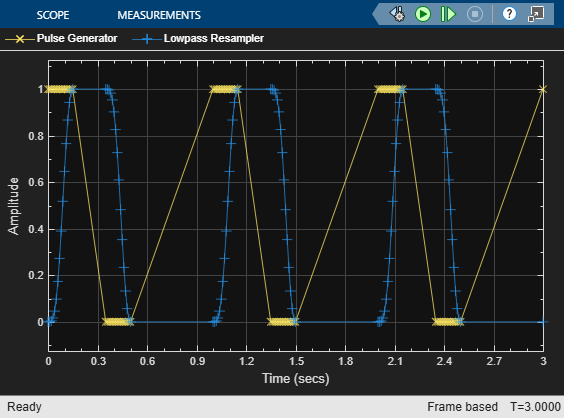# Define Output Samples in Lowpass Resampler

To demonstrate the variable step discrete behavior of the Lowpass Resampler block, open the model `LowpassResamplerOutputSampling`.

```model = 'LowpassResamplerOutputSampling'; open_system(model) ```The model consists of a Pulse Generator and a Lowpass Resampler. The pulse generator generates a pulse of 1 Hz with a duty cycle of 35%. The Pulse type is selected as `Time based`. In the Lowpass Resampler, the Output rise/fall time is set to `0.05` s, the Number of samples of delay is set to `1`, the Output sample time is set to `Variable step discrete`, and Samples out per rise/fall time is set to 5.

In the Model Settings, the Max step size of the solver is set to `3`, the simulation stop time. As a result, the only sample times are those generated by the model and the sample time display is readily understandable.

Run the simulation.

```sim(model) ```
```ans = Simulink.SimulationOutput: tout: [97x1 double] SimulationMetadata: [1x1 Simulink.SimulationMetadata] ErrorMessage: [0x0 char] ```To demonstrate the fixed step discrete behavior of the Lowpass Resampler block, set the Output sample time is set to `Fixed step discrete`. Rerun the simulation and observe the additional samples produced by the model.

```set_param([model '/Lowpass Resampler'],'OutputTsType','Fixed step discrete'); sim(model) ```
```ans = Simulink.SimulationOutput: tout: [301x1 double] SimulationMetadata: [1x1 Simulink.SimulationMetadata] ErrorMessage: [0x0 char] ```